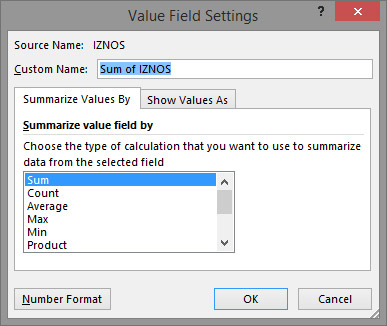# Value Field Settings

By dragging the dimensions with numeric values into the Values field, the automatic aggregation of data is performed. Applied mathematical operation can be: SUM, COUNT, AVERAGE … Sometimes there is a need to see a percentage of the value in relation to the total sum, a cumulative value increase (Running Total) or a different view instead of simple aggregation. The following text will be about how to change the Value Field Settings.

If we create a Pivot table and further drag ITEM NAME into Rows, and AMOUNT into Columns, we’ll get a common Pivot table report. Dragging dimensions into Values field implies aggregation of data, commonly by SUM function. If we want to change a function which is used to perform data aggregation we should start Field Settings option on Analyze ribbon. The Value Field Settings dialog will open, which contains two tabs: Summarize Values By and Show Values As.First tab contains a list of functions by which we can perform aggregation of data: SUM, AVERAGE, COUNT, MAX, MIN, PRODUCT etc. By choosing function other than SUM we’ll change the way of aggregation. For example, by choosing AVERAGE we’ll calculate average value of AMOUNT.

At the end of dialog box there is a Number Format button. Clicking on this button opens the Format Cells dialog box where you can format the display mode of values. This is a much more successful way of formatting than doing it directly within the Pivot table.

Other tab Show Values As has default value No Calculation. By changing default value we’ll change the way that values are being shown. Available options are:

• % Of Grand Total– percentage value relative to the grand total
• % Of Column Total– percentage value relative to the column total
• % Of Row Total– percentage value relative to the row total
• % Of – percentage value relative to the previous, next, or other value from the set
• % of Parent Row Total– percentage value in relation to the superior row
• % of Parent Column Total– percentage value in relation to the superior column
• % of Parent Total– percentage value in relation to the superior set member
• Difference From– difference in relation to the stated value
• % Difference From– difference in relation to the stated value in percents
• Running Total In– cumulative amount
• % Running Total In– procentuelna kumulativna suma
• Rank Smallest To Largest– ranking values by the rule that the smallest value is 1, and each has a higher value rank more
• Rank Largest To Smallest– value ranking according to the rule that the highest value is 1, and each smaller has the rank value more
• Index– calculates and displays the value of the index according to the formula: (value * total sum) / (total sum per row * total sum per column)

When dragging the dimensions into a Values field, it is possible to drag a dimension several times. So, for example, we can drag AMOUNT dimension twice. First one should be an amount. For a repeat value, for example, we choose to display % Of Grand Total and we will see in the report a comparative representation of the value and its percentage share in the total sum.